Dear Students, you have learnt about reflection of light, mirror, image formation by mirror, refraction of light and refractive index of a mdium. Now, let’s know what happen when a ray of light passes throuh rectangular glass slab ? What happen when a ray of ligt passes through a glass prism and why ? Dear students, if you have not learn said topics, then immediately visit the following links to study. https://www.mindshut.com/reflection-of-light-class-10-physics/

https://www.mindshut.com/mirror-and-terms-related-to-spherical-mirror-class-10-physics/

https://www.mindshut.com/refraction-of-light-class-10-physics/

## Refraction through Rectangular Glass Slab :

We know that when a ray of light travels from one medium to another, it changes its path at the interface of two media. On going from rare medium to denser medium, it bents towards the normal at the separating surface of two media. We may observe that a ray of light gets refracted away from the normal when it passes from denser medium to rare medium. Now let’s observe the passing of ray of light from a rectangular glass slab.

In the above image we may observe that a monochromatic ligt ray is passing through a rectnangular glass slab. At first, it falls on a surface then it enters into the glass. That means, the ray is traveling from rare medium ( air ) to denser medium ( glass ) and hence it bents towards the normal. After that, it will emerge from glass that is it passes from denser medium ( glass ) to rare medium ( air ). Therefore, the emergent ray is away from the normal. Dear students…. Here one thing is to be noted that incident medium and emergent medium both are same ( air ) and emergent ray is parallel to the incident ray. This parallelism is due to the parallelism of incident surface and emergent surfaces.

Now let’s see how Snell’s Law will help to understand the refraction of light through a rectangular glass slab mathematically or theoritically.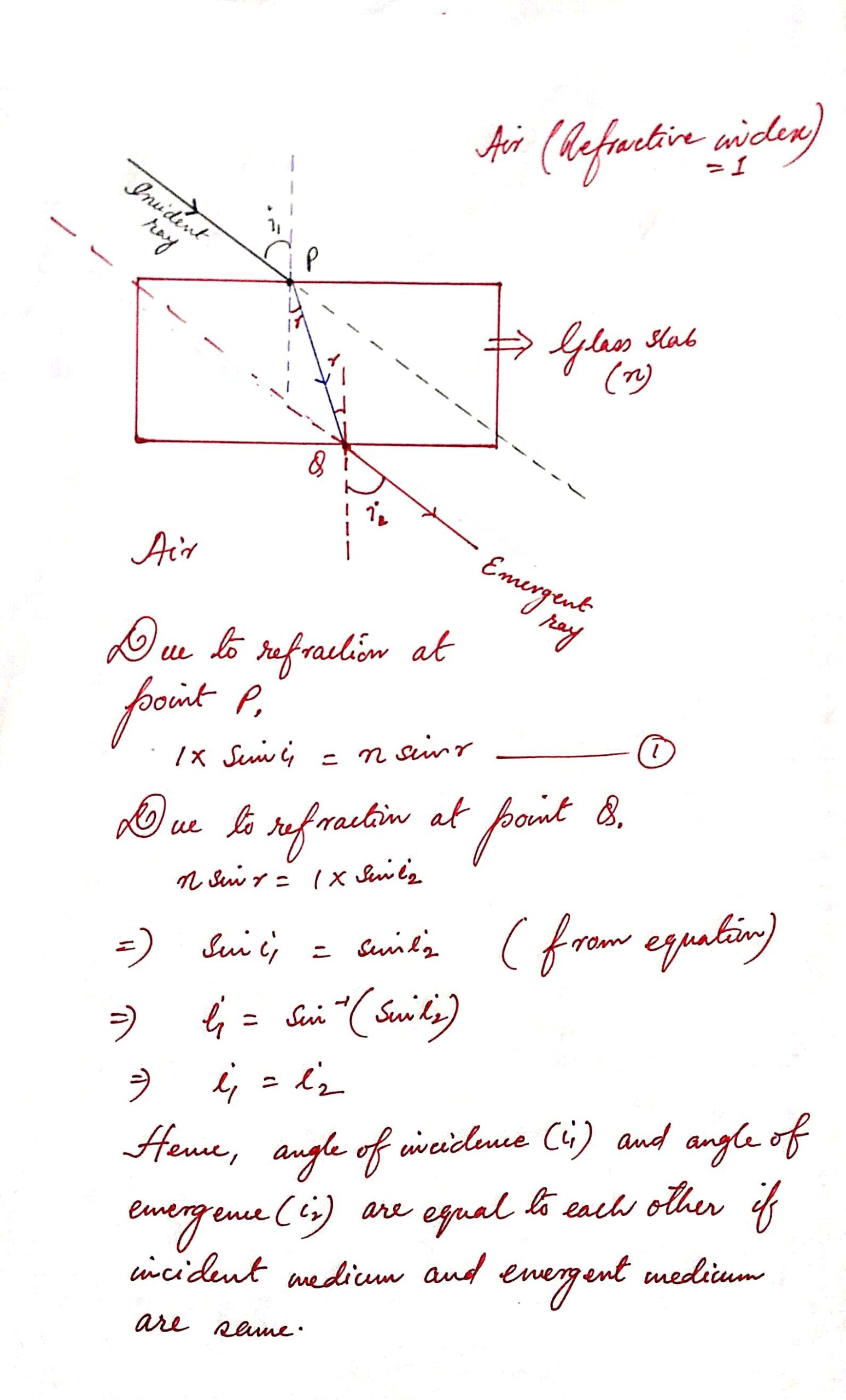### Conclusion :

1 ) Incident ray is parallel to emergent ray or vice-versa with a parallel shift.

2 ) The measurement of incident angle and emergent angle are equal to each other if incident medium and emergent medium will be the same.

Dear students….. Now let’s what happen when a ray of light passes through a Glass Prism. The following image demonstrates the passing of a monochromatic light through a glass prism.

## Refraction of Light through a Glass Prism :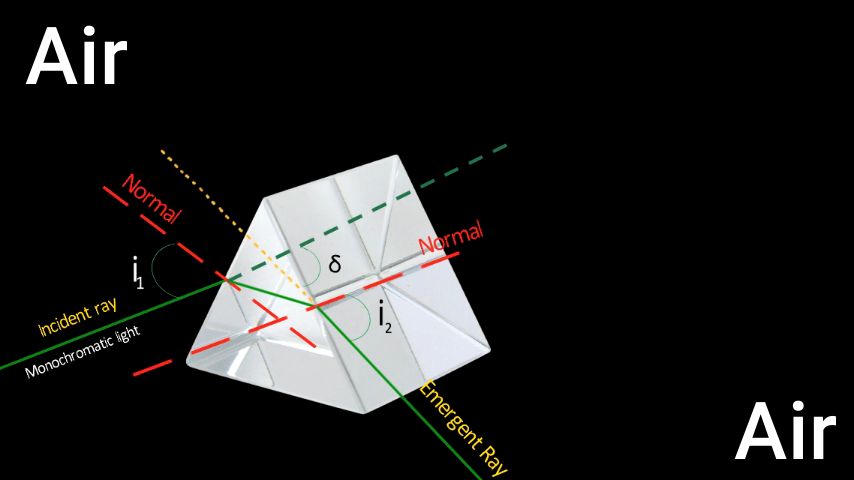Dear students….. Here we may observe that incident ray is NOT parallel to the emergent ray when a monochromatic light is passing through a glass prism. It deviates from its original path. Now let’s discuss what should be the mathematical expression for this deviation.

## Mathematical Expression for the Angle of Deviation :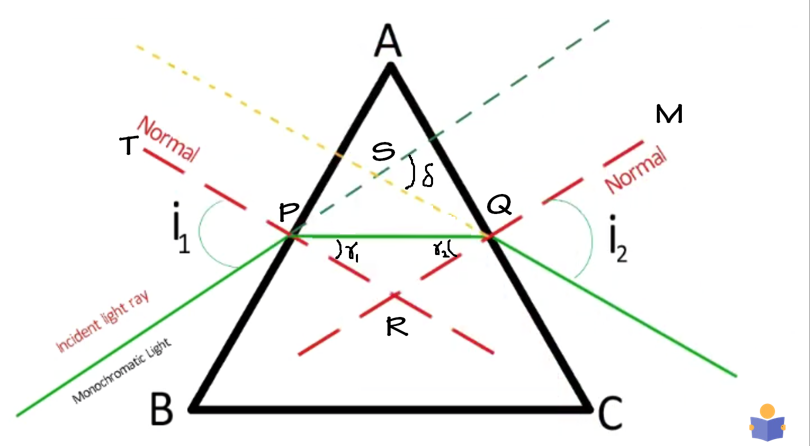Let i1 = incident angle

i2 = emergent angle

? = angle of deviation

r1 = angle of refraction at the surface AB.

r2 = angle of incidence at the surface AC.

A = angle of prism = PAQ = BAC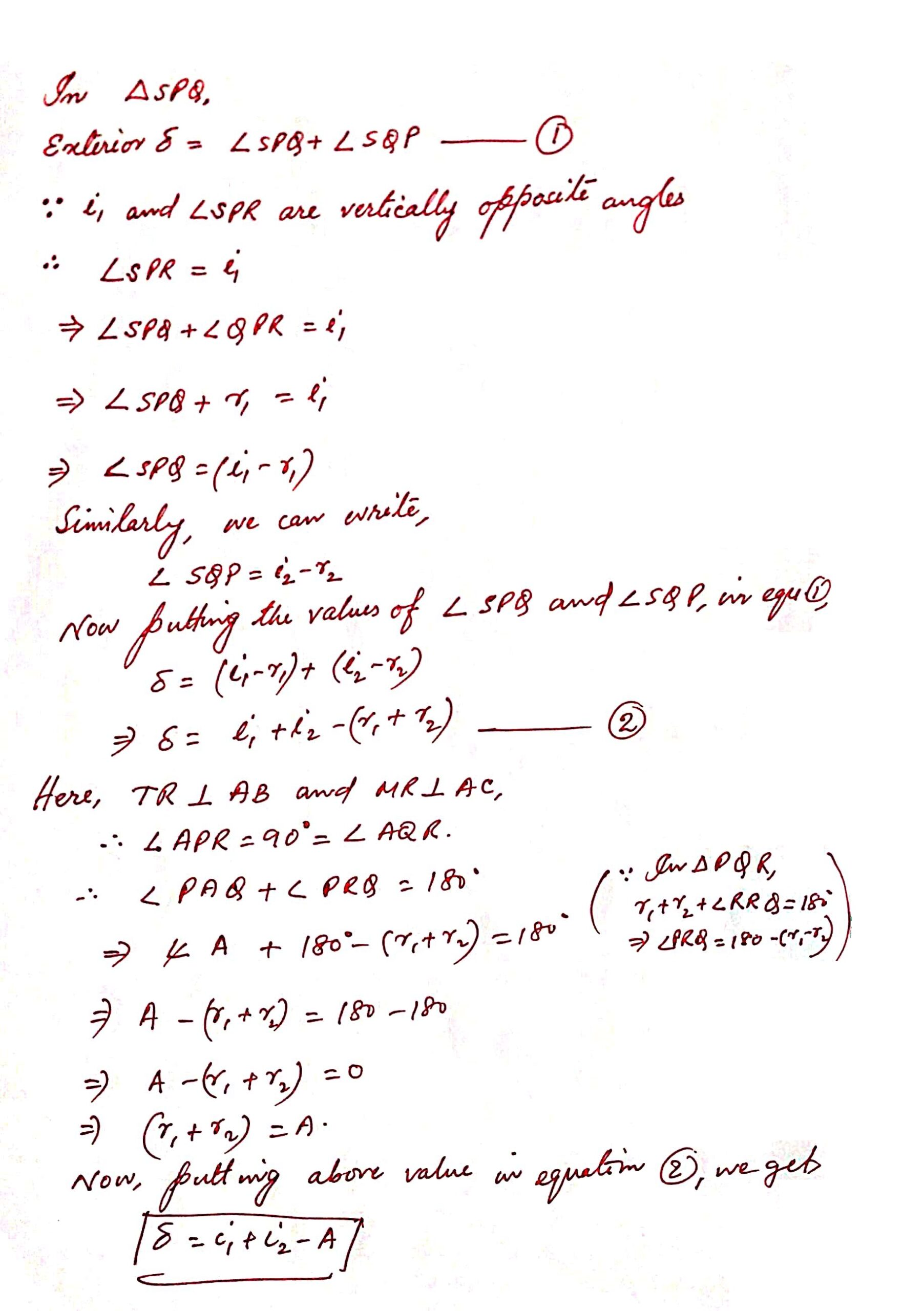Dear Students ….. You may think that angle of deviation depends on the value of angle of incidence, angle of emergence and angle of prism but the fact is very different. Let’s discuss the factors affecting the value of angle of deviation when a ray of light passes through a glass prism.

## Factors affecting Angle of Deviation :

Dear studnets …. after the experiment and observation, we have come to know that following are the factors that affects the value of angle of deviation when a monochromatic light passes through a glass prism.

1 ) Angle of Incidnence

2 ) Material of the Prism or Refractive Index.

3 ) Angle of Prism

4 ) Color of Light or Wavelenght of Light

## Dependence of Angle of Deviation on Angle of Incidence.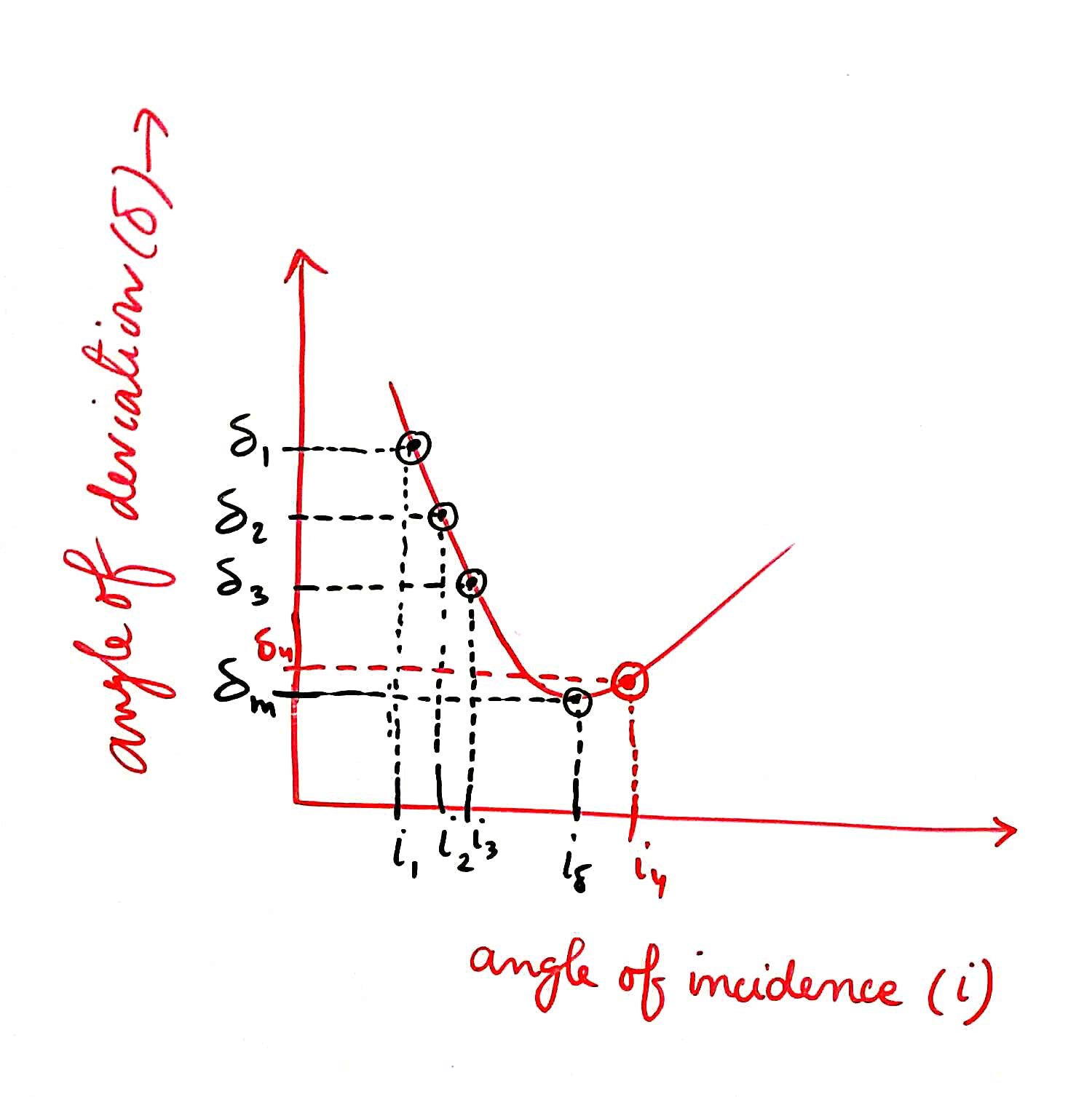Suppose we have data of angle of incidence and angle of deviation and if we plot these data on a graph sheet, we will get above graph. This graph clearly shows the relation between angle of incidnece and angle of deviation. At starting, if we increase the value of angle of incidnece, angle of deviation decreases. After reaching a specific value of angle of incidnece, the angle of deviation increasese with the increment of angle of incidence.

Thus i? is a particular value of angle of incidnece before and after that angle of deviation increases. The angle of deviation corresponding to the i? is called as minimum angle of deviation.

## Dependence of Angle of Deviation on Material of the Prism .

Refractive Index of a prism depends on the material of the prsim. The value of angle of deviation is directly proportional to the refractive index of the the material of the prism.

## Dependence of Angle of Deviation on Angle of Prism.

Angle of deviation is directly proportional to the angle of prism.

## Dependence of Angle of Deviation on Wavelength of Light.

As we know that refractive index is indirectly proportional to the wavelength of light and angle of deviation is directly proportional to the refractive index, consequently angle of deviation is indirectly proportional to the wavelength.

Greater the value of wavelength, lesser will be angle of deviation. As we know that red colour has maximum wavelength, refractive for red colour is minimum. Hence, angle of deviation will be minimum for red colour.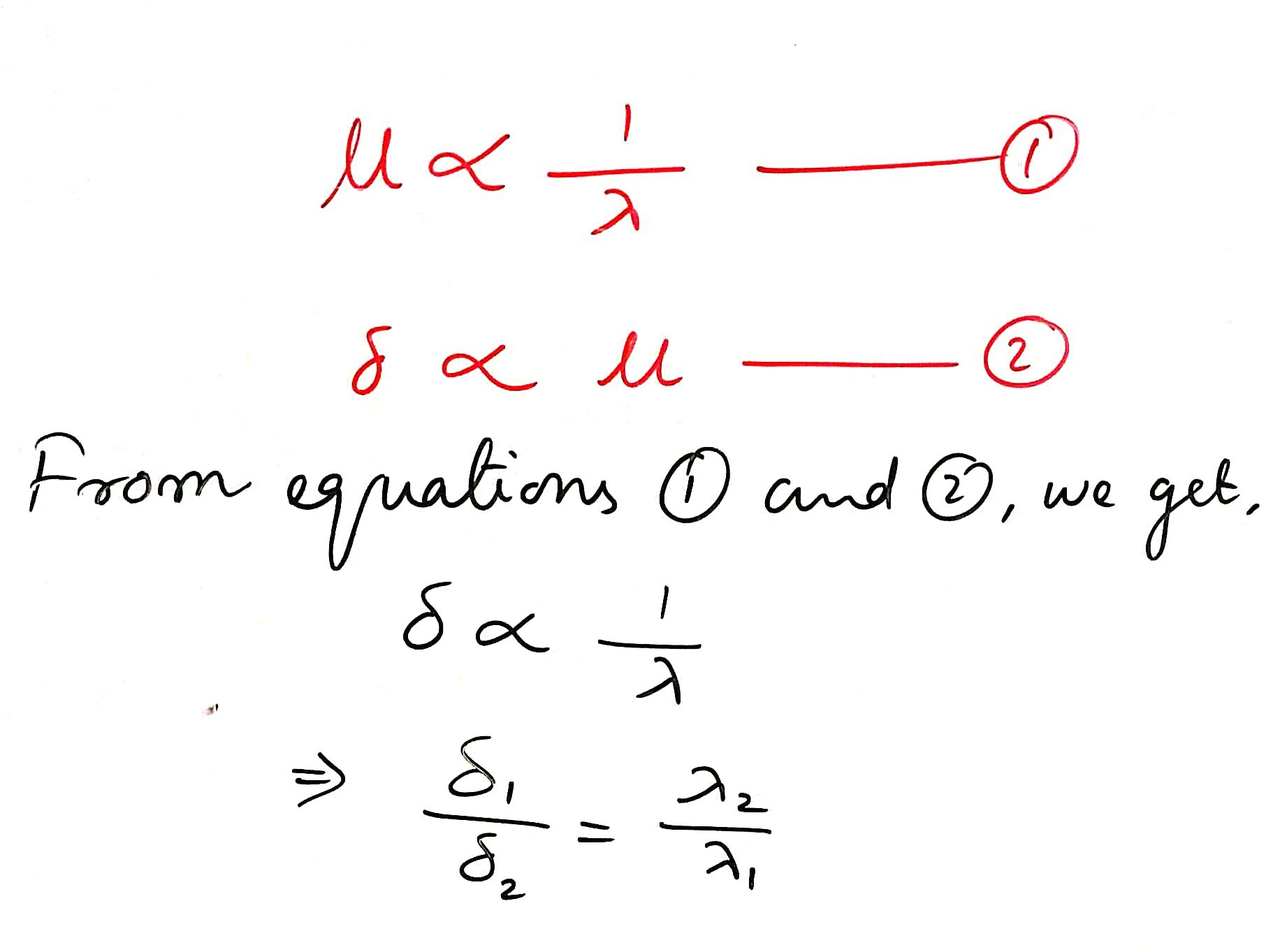Therefore, red colour is used for traffic signal or danger signal.